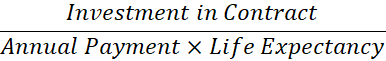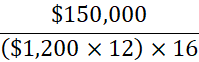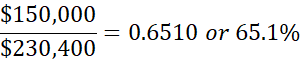This question is a part of BIF's Special Webinar series which was created to help students affected by the July 2020 CFP® Exam postponement to September 2020.

Ingrid, age 70, has owned a flexible premium deferred variable annuity for 10 years. She will begin annuity distributions this year. The total investment in Ingrid’s annuity is \$150,000. The anticipated monthly annuity payment is \$1,200 and her life expectancy (per IRS Table V) is 16 years.

Select the amount (rounded to the nearest dollar) of Ingrid’s annual annuity income that will be subject to taxation:

1. \$9,374
2. \$5,026
3. \$14,400
4. \$0

Instructor insight:

This problem can be solved by using the Exclusion Ratio formula:Ingrid’s total investment in the contract was \$150,000. This amount is divided by the annual annuity payments (\$1,200 x 12) times the life expectancy factor of 16.Therefore, 65.1% of each annuity payment will be excluded from taxation. The amount subject to tax can be found by subtracting the excluded percentage from 1:

1 – 0.651 = 0.349

Finally, the taxable percentage is multiplied by the total annual payments to solve for the taxable amount:

\$14,400 x 0.349 = \$5,026

CFP® Exam insight:

If annuity payments continue beyond the life expectancy noted on IRS Table V, all income generated from the annuity is considered taxable income.

Topics: Practice Questions